# Torque

11 de Mar de 2014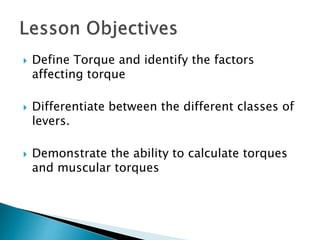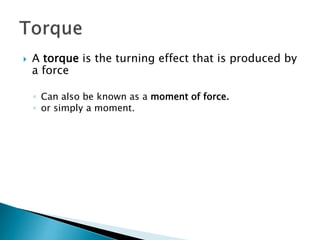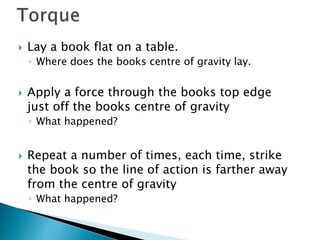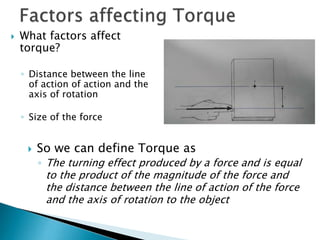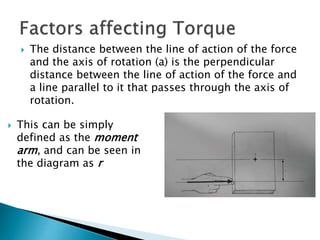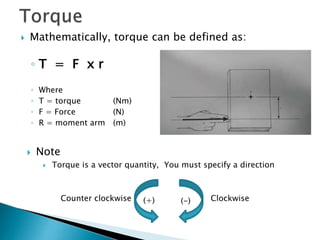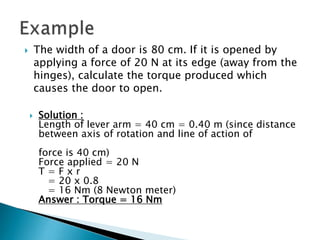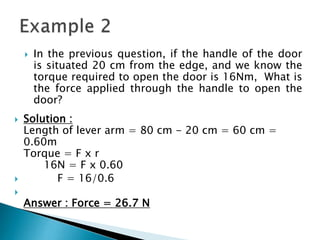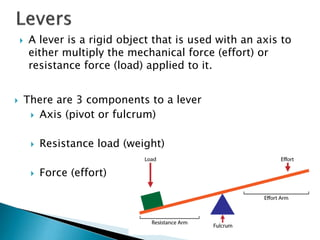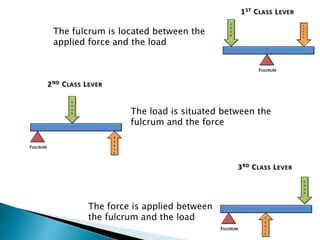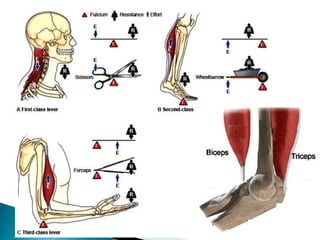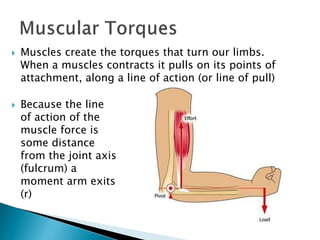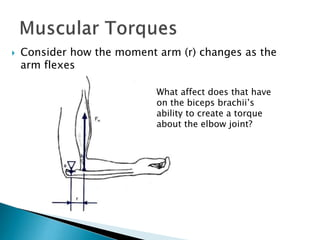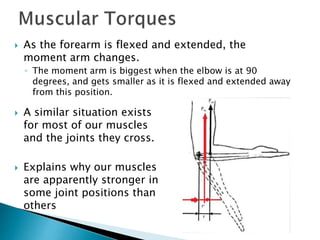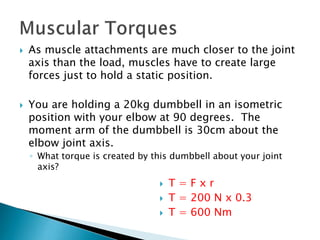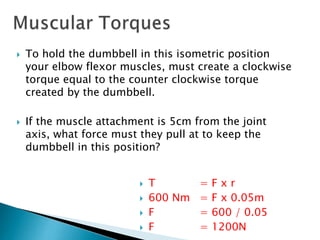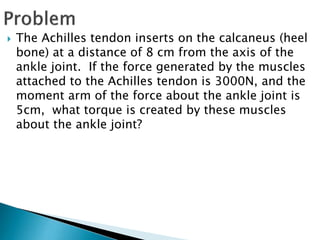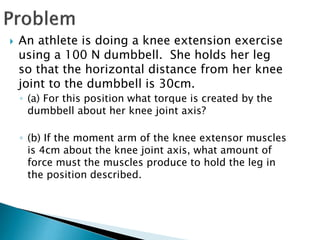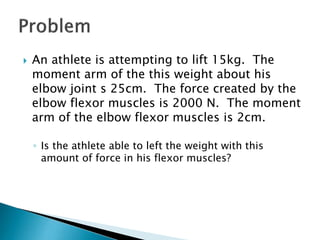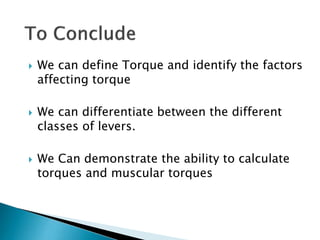1 de 21

### Torque

• 1. Torques and moments of force
• 2.  Define Torque and identify the factors affecting torque  Differentiate between the different classes of levers.  Demonstrate the ability to calculate torques and muscular torques
• 3.  A torque is the turning effect that is produced by a force ◦ Can also be known as a moment of force. ◦ or simply a moment.
• 4.  Lay a book flat on a table. ◦ Where does the books centre of gravity lay.  Apply a force through the books top edge just off the books centre of gravity ◦ What happened?  Repeat a number of times, each time, strike the book so the line of action is farther away from the centre of gravity ◦ What happened?
• 5.  What factors affect torque? ◦ Distance between the line of action of action and the axis of rotation ◦ Size of the force  So we can define Torque as ◦ The turning effect produced by a force and is equal to the product of the magnitude of the force and the distance between the line of action of the force and the axis of rotation to the object
• 6.  The distance between the line of action of the force and the axis of rotation (a) is the perpendicular distance between the line of action of the force and a line parallel to it that passes through the axis of rotation.  This can be simply defined as the moment arm, and can be seen in the diagram as r
• 7.  Mathematically, torque can be defined as: ◦ T = F x r ◦ Where ◦ T = torque (Nm) ◦ F = Force (N) ◦ R = moment arm (m)  Note  Torque is a vector quantity, You must specify a direction (+) (-)Counter clockwise Clockwise
• 8.  The width of a door is 80 cm. If it is opened by applying a force of 20 N at its edge (away from the hinges), calculate the torque produced which causes the door to open.  Solution : Length of lever arm = 40 cm = 0.40 m (since distance between axis of rotation and line of action of force is 40 cm) Force applied = 20 N T = F x r = 20 x 0.8 = 16 Nm (8 Newton meter) Answer : Torque = 16 Nm
• 9.  In the previous question, if the handle of the door is situated 20 cm from the edge, and we know the torque required to open the door is 16Nm, What is the force applied through the handle to open the door?  Solution : Length of lever arm = 80 cm - 20 cm = 60 cm = 0.60m Torque = F x r 16N = F x 0.60  F = 16/0.6  Answer : Force = 26.7 N
• 10.  A lever is a rigid object that is used with an axis to either multiply the mechanical force (effort) or resistance force (load) applied to it.  There are 3 components to a lever  Axis (pivot or fulcrum)  Resistance load (weight)  Force (effort)
• 11. The fulcrum is located between the applied force and the load The load is situated between the fulcrum and the force The force is applied between the fulcrum and the load
• 13.  Muscles create the torques that turn our limbs. When a muscles contracts it pulls on its points of attachment, along a line of action (or line of pull)  Because the line of action of the muscle force is some distance from the joint axis (fulcrum) a moment arm exits (r)
• 14.  Consider how the moment arm (r) changes as the arm flexes What affect does that have on the biceps brachii’s ability to create a torque about the elbow joint?
• 15.  As the forearm is flexed and extended, the moment arm changes. ◦ The moment arm is biggest when the elbow is at 90 degrees, and gets smaller as it is flexed and extended away from this position.  A similar situation exists for most of our muscles and the joints they cross.  Explains why our muscles are apparently stronger in some joint positions than others
• 16.  As muscle attachments are much closer to the joint axis than the load, muscles have to create large forces just to hold a static position.  You are holding a 20kg dumbbell in an isometric position with your elbow at 90 degrees. The moment arm of the dumbbell is 30cm about the elbow joint axis. ◦ What torque is created by this dumbbell about your joint axis?  T = F x r  T = 200 N x 0.3  T = 600 Nm
• 17.  To hold the dumbbell in this isometric position your elbow flexor muscles, must create a clockwise torque equal to the counter clockwise torque created by the dumbbell.  If the muscle attachment is 5cm from the joint axis, what force must they pull at to keep the dumbbell in this position?  T = F x r  600 Nm = F x 0.05m  F = 600 / 0.05  F = 1200N
• 18.  The Achilles tendon inserts on the calcaneus (heel bone) at a distance of 8 cm from the axis of the ankle joint. If the force generated by the muscles attached to the Achilles tendon is 3000N, and the moment arm of the force about the ankle joint is 5cm, what torque is created by these muscles about the ankle joint?
• 19.  An athlete is doing a knee extension exercise using a 100 N dumbbell. She holds her leg so that the horizontal distance from her knee joint to the dumbbell is 30cm. ◦ (a) For this position what torque is created by the dumbbell about her knee joint axis? ◦ (b) If the moment arm of the knee extensor muscles is 4cm about the knee joint axis, what amount of force must the muscles produce to hold the leg in the position described.
• 20.  An athlete is attempting to lift 15kg. The moment arm of the this weight about his elbow joint s 25cm. The force created by the elbow flexor muscles is 2000 N. The moment arm of the elbow flexor muscles is 2cm. ◦ Is the athlete able to left the weight with this amount of force in his flexor muscles?
• 21.  We can define Torque and identify the factors affecting torque  We can differentiate between the different classes of levers.  We Can demonstrate the ability to calculate torques and muscular torques